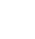# UDF Extract all comments from a cell

This UDF (User defined function) is designed to be pasted into a VB Module (ALT + F11) and will then become a normal everyday formula that you can use in Excel to get the comment text out of a particular cell.

There may be occasions when for instance a small database has been designed and lots of information has been stored under the comments (red cornered cell) but over time as a database increases in size, these comments can become lost in the data.

The example UDF below is used as a normal formula in Excel, referencing a cell which will return the comments in the cell where the formula was placed.

Use the following formula in a cell on a worksheet to get the comments stored in cell A1:

``` =GetCommentText(A1) ```

``` Function GetCommentText(rCommentCell As Range) Dim strGotIt As String On Error Resume Next strGotIt = WorksheetFunction.Clean _ (rCommentCell.Comment.Text) GetCommentText = strGotIt On Error GoTo 0 End Function ```